# CBSE Class 12 Board Exam Maths Paper 2018 2019 2020 With Solutions

Here we are providing CBSE Previous Year Question Papers Class 6 to 12 solved with soutions CBSE Class 12 Board Exam Maths Paper 2018 2019 2020 with Solutions Maths sample paper class 12, Maths previous year question paper class 12, cbse class 12 Maths sample paper, cbse class 12 Maths sample paper 2020, Maths sample paper class 12 2020, cbse sample paper 2020 class 12 Maths, class 12 Maths sample paper 20 Practice of previous year question papers and sample papers protects each and every student to score bad marks in exams.If any student of CBSE Board continuously practices last year question paper student will easily score high marks in tests. Fortunately earlier year question papers can assist the understudies with scoring great in the tests. Unraveling previous year question paper class 12 Maths is significant for understudies who will show up for Class 12 Board tests.

## Class 12 Subject Maths Paper Set 1 with Solutions

Section – A

Question numbers 1 to 10 are multiple choice questions. Select the correct option :

Question 1: (Marks 1)

If A is a square matrix of order 3, such that A (adj A) = 10 I, then |adj A| is equal to

(a) 1

(b) 10

(c) 100

(d) 101

Answer :

(c) 100

Question 2: (Marks 1)

If A is a 3 × 3 matrix such that |A| = 8, then |3A| equals.

(a) 8

(b) 24

(c) 72

(d) 216

Answer :

(d) 216

Question 3: (Marks 1)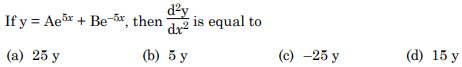Answer :

(a) 25y

Question 4: (Marks 1)Answer :Question 5: (Marks 1)Answer :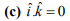Question 6: (Marks 1)Answer :Question 7: (Marks 1)Answer :

,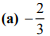Question 8: (Marks 1)

The graph of the inequality 2x + 3y > 6 is

(a) half plane that contains the origin.

(b) half plane that neither contains the origin nor the points of the line 2x + 3y = 6.

(c) whole XOY – plane excluding the points on the line 2x + 3y = 6.

(d) entire XOY plane.

Answer :

(b) half plane that neither contains the origin nor the points of the line 2x + 3y = 6.

Question 9: (Marks 1)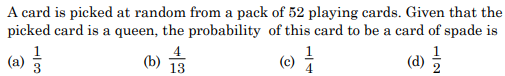Answer :Question 10: (Marks 1)

’,Answer :

(d) 1

Fill in the blanks in Questions from 11 to 15.

Question 11: (Marks 1)

A relation in a set A is called _______ relation, if each element of A is related to itself.

Answer :

reflexive

Question 12: (Marks 1)Answer :Question 13: (Marks 1)

,Answer :

2ab

Question 14: (Marks 1)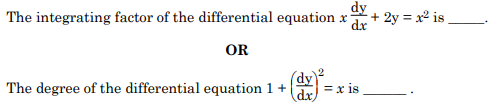Answer :

x2

Question 15: (Marks 1)

The vector equation of a line which passes through the points (3, 4, –7) and (1, – 1, 6) is _______.

OR

The line of shortest distance between two skew lines is _______ to both the lines.

Answer :

,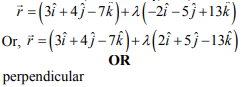Q. Nos. 16 to 20 are of very short answer type questions.

Question 16: (Marks 1)Answer :Question 17: (Marks 1)Answer :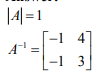Question 18: (Marks 1)

’,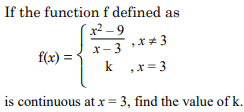Answer :Question 19: (Marks 1)Answer :Question 20: (Marks 1)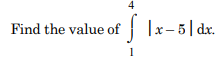Answer :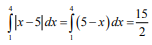Section – B

Q. Nos. 21 to 26 carry 2 marks each.

Question 21: (Marks 2)Answer :Question 22: (Marks 2)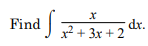Answer :Question 23: (Marks 2)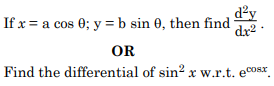Answer :Question 24: (Marks 2)Answer :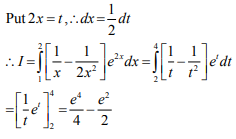Question 25: (Marks 2)Answer :Question 26: (Marks 2)

Given two independent events A and B such that P(A) = 0.3 and P(B) = 0.6, find P(A’ ⋂ B’)

Answer :

P (A’ ⋂ B’) = P (A’) P (B’)

= (0.7) (0.4) = 0.28

Section – C

Q. Nos. 27 to 32 carry 4 marks each.

Question 27: (Marks 4)Answer :Question 28: (Marks 4)Answer :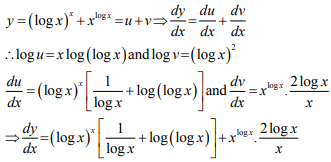Question 29: (Marks 4)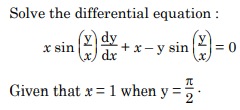Answer :Question 30: (Marks 4)Answer :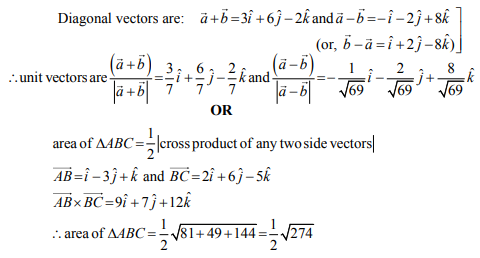Question 31: (Marks 4)

A company manufactures two types of novelty souvenirs made of plywood. Souvenirs of type A requires 5 minutes each for cutting and 10 minutes each for assembling. Souvenirs of type B require 8 minutes each for cutting and 8 minutes each for assembling. Given that total time for cutting is 3 hours 20 minutes and for assembling 4 hours. The profit for type A souvenir is ₹ 100 each and for type B souvenir, profit is ₹ 120 each. How many souvenirs of each type should the company manufacture in order to maximize the profit ? Formulate the problem as an LPP and solve it graphically.

Answer :

,Question 32: (Marks 4)

Three rotten apples are mixed with seven fresh apples. Find the probability distribution of the number of rotten apples, if three apples are drawn one by one with replacement. Find the mean of the number of rotten apples.

OR

In a shop X, 30 tins of ghee of type A and 40 tins of ghee of type B which look alike, are kept for sale. While in shop Y, similar 50 tins of ghee of type A and 60 tins of ghee of type B are there. One tin of ghee is purchased from one of the randomly selected shop and is found to be of type B. Find the probability that it is purchased from shop Y

Answer :Section – D

Q. 33 to 36, carry 6 marks each.

Question 33: (Marks 6)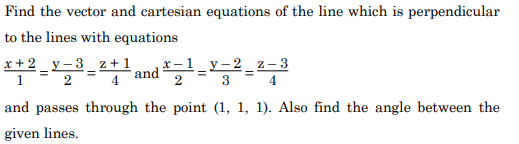Answer :Question 34: (Marks 6)Answer :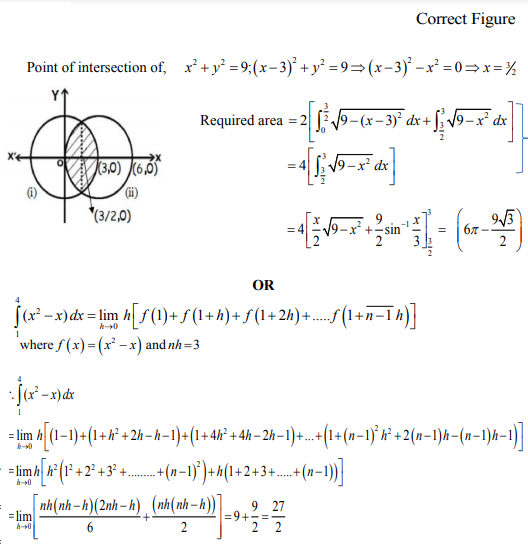Question 35: (Marks 6)

Find the minimum value of (ax + by), where xy = c2. .

Answer :Question 36: (Marks 6)Answer :☞ Click here for privious year Question papers

## Maths sample paper class 12, Maths previous year question paper class 12, cbse class 12 Maths sample paper, cbse class 12 Maths sample paper 2020, Maths sample paper class 12 2020, cbse sample paper 2020 class 12 Maths, class 12 Maths sample paper 2020, class 12 important questions Maths, cbse class 12 board exam Maths paper, Maths previous year question papers class 12 with solutions, Maths sample paper class 12 2019, cbse class 12 Maths question paper 2017 solved pdf, cbse class 12 Maths question paper 2018, class 12 Maths paper 2019, Maths question paper for class 12, cbse class 12 Maths paper 2019

### NCERT Books Free Pdf Download for Class 5, 6, 7, 8, 9, 10 , 11, 12 Hindi and English Medium

 Mathematics Biology Psychology Chemistry English Economics Sociology Hindi Business Studies Geography Science Political Science Statistics Physics Accountancy

## Please Share this webpage on facebook, whatsapp, linkdin and twitter.

Copyright @ ncerthelp.com A free educational website for CBSE, ICSE and UP board.